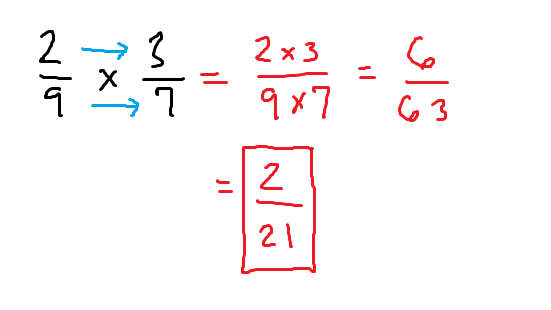﻿ Multiplying fractions and cross-cancelling - MathBootCamps

# Multiplying fractions and cross-cancelling

Fractions can seem to be a complicated idea in math. But multiplying fractions turns out to be one of the easier things you can do when you are working with fractions! One reason is that when multiplying fractions, you do not have to worry about common denominators. Instead, you always apply the same rule: multiply straight across. You can see this in the examples below or you can scroll down for a video example.

## Some examples of multiplying fractions

Let’s look at our first example. Here, we are finding the product: $$\dfrac{3}{4} \times \dfrac{1}{2}$$Notice that the answer was already simplified and that even though the denominators were not the same, you could still just multiply across. In the next example, we will also use that rule, but the answer has to be simplified.There is a shortcut that we can use when this happens called cross-cancelling.

## Cross-cancelling: simplifying before multiplying fractions

Let’s use the same example as before. Notice that the 3 and the 9 both share a factor of 3 since $$3 = 3 \times 1$$ and $$9 = 3 \times 3$$. Because of this, we can cross cancel before we multiply.This is much easier! It even works when the fractions are a bit more complicated, as in the example below where we are finding:

$$\dfrac{11}{12} \times \dfrac{26}{55}$$

Here, 11 and 55 both share a factor of 11 since $$11 \times 1 x 11$$ and $$55 = 5 \times 11$$. Also, 26 and 12 both share a factor of 2 since $$26 = 2 \times 13$$ and $$12 = 2 \times 6$$.## Video – how to multiply two fractions

The video below goes into a little bit of the ideas behind multiplying two fractions and then shows some examples, including examples where you can cross-cancel.

## Summary

When adding or subtracting fractions, the process is different depending on whether the denominators are the same or different. However, when multiplying or even dividing fractions, this is no longer a concern. Instead, you multiply the two numbers in the numerators and multiply the two numbers in the denominators. To save work later, always remember to check if you can cross-cancel.## Subscribe to our Newsletter!

We are always posting new free lessons and adding more study guides, calculator guides, and problem packs.

Sign up to get occasional emails (once every couple or three weeks) letting you know what's new!